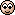# Magnetic susceptibility of Superconductor

lepori
as we know, B=0 in superconductors, because of Messner effect.

B0(H+M)
=>
H=-M
=>
χ=-1
in CGS units:
B=H+4πM
=> H=-4πM
χ=-1/4π
---
question:

why χ=-0.01 not χ=-1/4π for 100% superconductors?